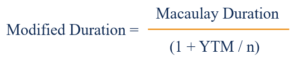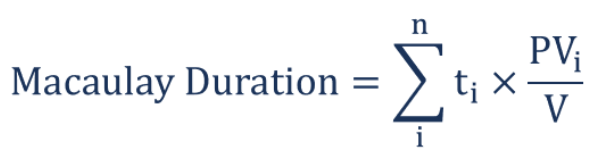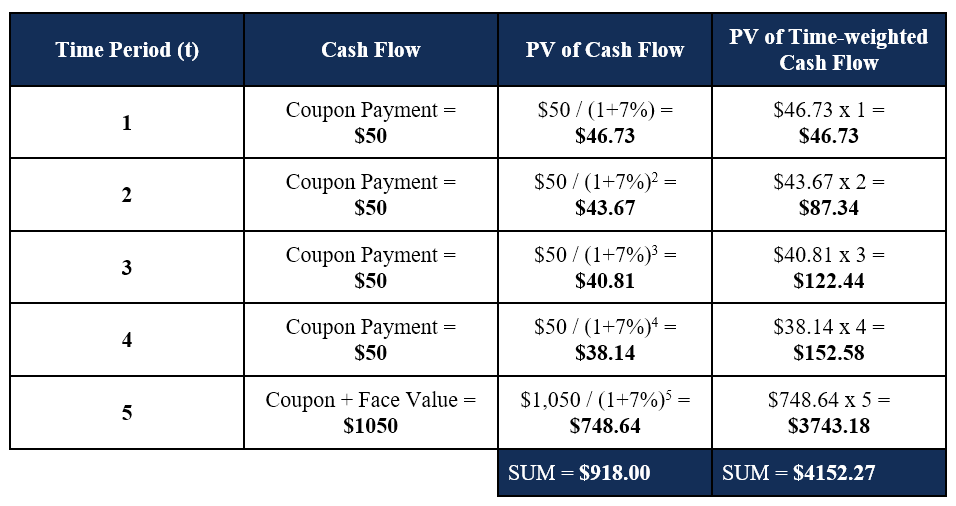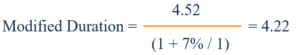# Modified Duration

The change in the value of a security due to a change in interest rates

## What is Modified Duration?

Modified duration, a formula commonly used in bond valuations, expresses the change in the value of a security due to a change in interest rates. In other words, it illustrates the effect of a 100-basis point (1%) change in interest rates on the price of a bond.

Modified duration illustrates the concept that bond prices and interest rates move in opposite directions – higher interest rates lower bond prices, and lower interest rates raise bond prices.

### Formula for Modified Duration

The formula for modified duration is as follows:Where:

• Macaulay Duration is the weighted average number of years an investor must maintain his or her position in the bond where the present value (PV) of the bond’s cash flow equals the amount paid for the bond. In other words, it is the time it would take for an investor to retrieve the money initially invested in the bond
• YTM stands for Yield to Maturity and is the total return on a bond if held until maturity
• n is the number of coupon periods per year.

### Understanding the Macaulay Duration

In order to arrive at the modified duration of a bond, it is important to understand the numerator component – the Macaulay duration – in the modified duration formula.

The Macaulay duration is the weighted average of time until the cash flows of a bond are received. In layman’s term, the Macaulay duration measures, in years, the amount of time required for an investor to be repaid his initial investment in a bond. A bond with a higher Macaulay duration will be more sensitive to changes in interest rates.

The formula for Macaulay duration is as follows:Where:

• ti is the time period
• PViis the present value of the time-weighted cash flow
• V is the present value of all cash flow.

Below is an example of calculating Macaulay duration on a bond.

### Example of Macaulay Duration

Tim holds a 5-year bond with a face value of \$1,000 and an annual coupon rate of 5%. The current rate of interest is 7%, and Tim would like to determine the Macaulay duration of the bond. The calculation is given below:The Macaulay duration for the 5-year bond is calculated as \$4152.27 / \$918.00 = 4.52 years.

### Putting it Together

Now that we understand and know how to calculate the Macaulay duration, we can determine the modified duration.

Using the example above, we simply insert the figures into the formula to determine the modified duration:The modified duration is 4.22.

### Interpreting the Modified Duration

How do we interpret the result above? Recall that modified duration illustrates the effect of a 100-basis point (1%) change in interest rates on the price of a bond.

Therefore,

• If interest rates increase by 1%, the price of the 5-year bond will decrease by 4.22%.
• If interest rates decrease by 1%, the price of the 5-year bond will increase by 4.22%.

The modified duration provides a good measurement of a bond’s sensitivity to changes in interest rates. The higher the Macaulay duration of a bond, the higher the resulting modified duration and volatility to interest rate changes.